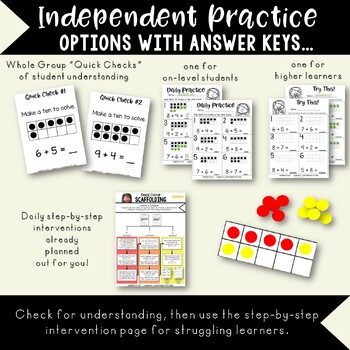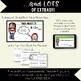# Distance Learning* Addition to 20 Common Core Easy Lessons for Home or SchoolResource Type
File Type

Zip

(141 MB|N/A)
Standards
Also included in:
1. This bundle contains all three of Lola's Addition to 20 units- includes 10 guided practice PowerPoint lessons, 10 differentiated guided math lessons, unit assessments, daily practice pages, daily enrichment pages, daily quick checks, and 8 centers and review games, and one set of Boom Cards!As a Bun
\$25.00
\$22.50
Save \$2.50
• Product Description
• Standards

These lessons do all of the teaching for you, so they are perfect for sending home to parents to continue the learning. Just run each lesson as a slideshow, and Lola will teach your students all about addition! These lessons are very hands-on, so students will need some sort of writing utensil (paper and pencil will work if they don't have a whiteboard at home) and some simple manipulatives to interact with the lessons.

**Common Core Aligned!** This bundle includes 10 interactive PowerPoint guided practice lessons to keep students engaged and learning! These lessons use number lines to 20, open number lines, number bond mats with cubes, ten frames and dry erase boards to work hands-on along with Lola.

Formerly associated with a single math curriculum, these lessons have been revamped to follow along with any Common Core Math boxed curriculum or stand alone for quality instruction!

Kids love the characters and familiar scenarios, and teachers love

how all the work is done for them. Just play each lesson as a slideshow and encourage student participation as you click through the animations!

Here are the lessons included in this bundle-

Lola Counts On to 20 with a Number Line

Lola Counts On with an Open Number Line

Lola Counts On to 20

Lola Uses Bigger Doubles

Lola Uses Doubles Plus One

Lola Practices Making 10 to Add

Lola Breaks It Down

Lola Solves Addition Story Problems to 20

Lola Finds Mistakes

WANT THE MATCHING GUIDED MATH UNIT?

Lola's Guided Math- Addition to 20

WANT THE MATCHING CENTERS?

Lola & Friends Math Centers- Addition to 20

LOVE LOLA???

WANT TO GET ACCESS TO ALL THE RESOURCES FOR THE BEST PRICE POSSIBLE?

CHECK OUT THIS FREEBIE: Everything Lola Membership Preview

This math lesson bundle is a part of the larger set-

Lola's Year-Long Mega Bundle of Guided Practice Common Core Math Lessons

If you have purchased this Mega Bundle, you do NOT need to purchase this unit!

If you are considering purchasing this bundle and are new to Lola's lessons, here are some freebie lessons you can try out:

Lola's Math Lesson Lessons- FREE

If you are looking for daily practice pages and small group lessons to go with these PowerPoint lessons, you may be interested in:

Addition to 20 Guided Math & Daily Practice

Check out the other units in this series:

Early Number Sense (10 lessons)

Numbers to 120 (10 lessons)

Subtraction from 10 (10 lessons)

Commutative Property to 10 (5 lessons)

Place Value (10 lessons)

Extra Practice with Story Problems (5 lessons)

Subtraction from 20 (10 lessons)

Commutative Property to 20 (5 lessons)

Comparing Numbers (10 lessons)

Equal Equations (5 lessons)

Data (5 lessons)

Measurement (5 lessons)

Time (5 lessons)

Shapes (10 lessons)

Fractions (5 lessons)

Two-Digit Subtraction (10 lessons)

***************************************************************************

Customer Tips:

How to get TPT credit to use on future purchases:

• Please go to your My Purchases page (you may need to login). Beside each purchase you'll see a Provide Feedback button. Simply click it and you will be taken to a page where you can give a quick rating and leave a short comment for the product. Each time you give feedback, TPT gives you feedback credits that you use to lower the cost of your future purchases. I value your feedback greatly as it helps me determine which products are most valuable for your classroom so I can create more for you. ☺

Be the first to know about my new discounts, freebies and product launches:

• Look for the green star next to my store logo and click it to become a follower. Voila! You will now receive email updates about this store. ☺

***************************************************************************

Determine the unknown whole number in an addition or subtraction equation relating three whole numbers. For example, determine the unknown number that makes the equation true in each of the equations 8 + ? = 11, 5 = ▯ - 3, 6 + 6 = ▯.
Add and subtract within 20, demonstrating fluency for addition and subtraction within 10. Use strategies such as counting on; making ten (e.g., 8 + 6 = 8 + 2 + 4 = 10 + 4 = 14); decomposing a number leading to a ten (e.g., 13 - 4 = 13 - 3 - 1 = 10 - 1 = 9); using the relationship between addition and subtraction (e.g., knowing that 8 + 4 = 12, one knows 12 - 8 = 4); and creating equivalent but easier or known sums (e.g., adding 6 + 7 by creating the known equivalent 6 + 6 + 1 = 12 + 1 = 13).
Relate counting to addition and subtraction (e.g., by counting on 2 to add 2).
Understand subtraction as an unknown-addend problem. For example, subtract 10 – 8 by finding the number that makes 10 when added to 8.
Apply properties of operations as strategies to add and subtract. If 8 + 3 = 11 is known, then 3 + 8 = 11 is also known. (Commutative property of addition.) To add 2 + 6 + 4, the second two numbers can be added to make a ten, so 2 + 6 + 4 = 2 + 10 = 12. (Associative property of addition.)
Total Pages
N/A
N/A
Teaching Duration
2 Weeks
Report this Resource to TpT
Reported resources will be reviewed by our team. Report this resource to let us know if this resource violates TpT’s content guidelines.